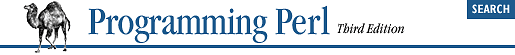## 6.3. Passing References

If you want to pass more than one array or hash into or out of a function, and you want them to maintain their integrity, then you'll need to use an explicit pass-by-reference mechanism. Before you do that, you need to understand references as detailed in Chapter 8, "References". This section may not make much sense to you otherwise. But hey, you can always look at the pictures...

Here are a few simple examples. First, let's define a function that expects a reference to an array. When the array is large, it's much faster to pass it in as a single reference than a long list of values:

```\$total = sum ( \@a );

sub sum {
my (\$aref)  = @_;
my (\$total) = 0;
foreach (@\$aref) { \$total += \$_ }
return \$total;
}```
Let's pass in several arrays to a function and have it pop each of them, returning a new list of all their former last elements:
```@tailings = popmany ( \@a, \@b, \@c, \@d );

sub popmany {
my @retlist = ();
for my \$aref (@_) {
push @retlist, pop @\$aref;
}
return @retlist;
}```

Here's how you might write a function that does a kind of set intersection by returning a list of keys occurring in all the hashes passed to it:

```@common = inter( \%foo, \%bar, \%joe );
sub inter {
my %seen;
for my \$href (@_) {
while (my \$k = each %\$href ) {
\$seen{\$k}++;
}
}
return grep { \$seen{\$_} == @_ } keys %seen;
}```
So far, we're just using the normal list return mechanism. What happens if you want to pass or return a hash? Well, if you're only using one of them, or you don't mind them concatenating, then the normal calling convention is okay, although a little expensive.

As we explained earlier, where people get into trouble is here:

`(@a, @b) = func(@c, @d);`
or here:
`(%a, %b) = func(%c, %d);`
That syntax simply won't work. It just sets @a or %a and clears @b or %b. Plus the function doesn't get two separate arrays or hashes as arguments: it gets one long list in @_, as always.

You may want to arrange for your functions to use references for both input and output. Here's a function that takes two array references as arguments and returns the two array references ordered by the number of elements they have in them:

```(\$aref, \$bref) = func(\@c, \@d);
print "@\$aref has more than @\$bref\n";
sub func {
my (\$cref, \$dref) = @_;
if (@\$cref > @\$dref) {
return (\$cref, \$dref);
} else {
return (\$dref, \$cref);
}
}```
For passing filehandles or directory handles into or out of functions, see the sections "Filehandle References" and Section 6.2.5, "Symbol Table References" in Chapter 8, "References".